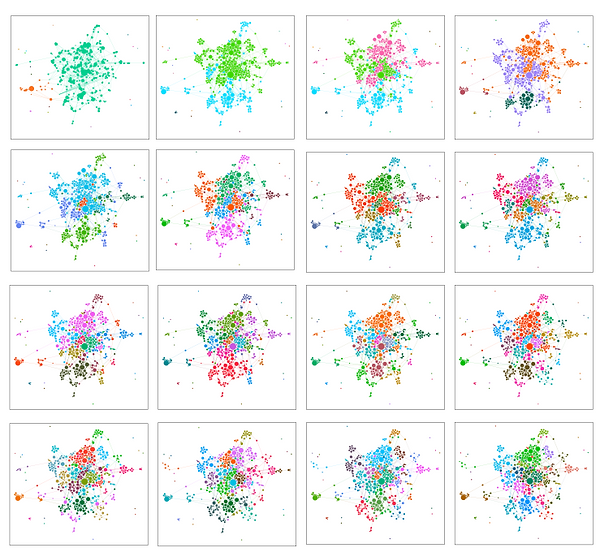top of page

# Quantitative MethodsCitation network analyses can take references (e.g., this present analysis; Nylander, Österlund, & Fejes, 2018), authors (e.g., ISI, 1981), or journals (e.g., Bruce et al., 2017; Wang & Bowers, 2016) as their unit of analysis. Depending on the unit of analysis, but regardless of the software package chosen, citation network analyses take one of three forms: coupling, mapping, or clustering. Coupling analyses connect two nodes whenever they share a reference (co-citation coupling), share keywords (co-classification coupling), share words within the title/abstract/body (co-word coupling), share an author (co-author), or are cited together by another article (co-citation coupling). I have chosen not to undertake a coupling analysis since it reduces the complexity of the network by approximating article content by its keywords (co-classification coupling), the words used in the title/abstract/text (co-word coupling), or its author (co-author coupling). Instead, I have chosen to perform a citation analysis directly where the data remains the complete list of every reference of every included article.

Layout analyses use mapping algorithms to calculate a placement for nodes on a map so that relative position of nodes encodes information about the relationships between them. For example, Fruchterman and Reingold’s (1991) novel force-directed layout algorithm (called Fruchterman-Reingold) treats nodes as electrons, whose physical tendency is to repel each other, and the edges between nodes as springs, whose physical tendency is to contract and attract each other. As shown in Figure 1, electron repulsion is governed by Coulomb’s Law and the force repelling nodes decreases as the distance between them increases. Similarly, Hooke’s Law governs spring contraction and the force contracting the spring increases as the distance between the ends of the spring increases. Between any two nodes, then, there is a force that increases as the distance increases and a force that decreases as the distance increases so that there is guaranteed to be a point at which these two forces are at equilibrium. Fruchterman-Reingold calculates these spring and electron forces for every node in a system in discrete steps. After a first calculation is made, the forces between nodes and on springs, are recalculated, continuing until a stable state is found (i.e., the next step returns a result sufficiently close to the previous). Jacomy, Venturini, Heymann, and Bastian updated (2014) Fruchterman and Reingold’s algorithm to a continuous model (ForceAtlas2). With this update, ForceAtlas2, instead of presenting snapshots of the map that successively approach the final state, presents a fluid animation and transformation from the initial to final state. Fruchterman and Reingold showed that such a force-directed algorithm encodes information about the nodes: articles of a similar topic attract each other and articles with vastly different topics repel (1991). It is for this reason that I chose to use a force-directed algorithm to develop the maps of the JRME, flm, and Educational Studies in Mathematics (ESM): articles of similar topic will be placed near each other. Furthermore, both Fruchterman-Reingold and ForceAtlas2 are included in Gephi.

To identify the conversation groups, then, I use a well-documented community finding algorithm (i.e., Louvain Modularity) to find densely connected subsets of articles. These densely connected subsets correspond to the conversation groups within the field of mathematics education research. For example, this algorithm identifies one cluster of research where scholars are studying computer programming in mathematics education, and other clusters group those interested in equity, discourse, problem solving, psychology of learning, pedagogical content knowledge, international comparative assessment, and more. Clustering algorithms are quantitative calculations that are independent of the layout of a citation network. While clustering algorithms do not consider the distance between two nodes, they do, however, consider on the number of edges between nodes. For example, Newman (2006) discusses the use of modularity to study the relationship between nodes and returns subsets of nodes which are densely connected, or in other words, clusters of articles that are heavily cross-citing the same literature.

The time to calculate every possible division into modularity classes, since it involves considering all possible subgroups of nodes and counting the number of edges contained in each group, is not computationally feasible on a large citation network in that it would take too long to complete the computation. Blondel, Guillaume, Lambiotte, and Lefebvre (2008), researchers at the Université catholique de Louvain, addressed this computational limit and modified Newman’s algorithm to quickly calculate modularity in large networks. This modified algorithm, called Louvain modularity, improves Newman’s algorithm by beginning with a random subdivision of nodes and by calculating the benefit of adding or removing a given node. If the benefit is not sufficiently large, the change in layout is not made. The algorithm runs from several different starting conditions and returns the result that is most modular. The Louvain Modularity algorithm is included in Gephi and is used to identify the clusters of research in each map. As shown by Waltman, van Eck, and Noyons (2010), the articles that ForceAtlas2 places near each other spatially are also clustered into the same modularity class by the Louvain Modularity algorithm. This successive process of dividing a network into more modularity classes until no additional benefit is achieved is shown in Figure 1 below. First, the JRME 1970s network is divided into two groups, then, looking across each row and across successive rows, the final result shows the network divided into 18 groups.

Figure 1. The result of successive subdivisions by the Louvain modularity algorithm from the JRME 1970s.bottom of page